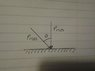# Determine Radiation pressure at angle given Perpendicular Pressure

• MrMoose
In summary, the radiation pressure exerted on a flat, totally reflecting surface of area A when the laser beam is angled at an angle θ is given by Pr[θ] = (Cos(θ))^2 * Pr[p], where Pr[p] is the pressure that would be exerted if the beam were perpendicular to the surface. This is due to the fact that the plate intercepts fewer light rays at an angle, resulting in a decrease in intensity and radiation pressure per unit of intensity, both of which are proportional to Cos(θ).

## Homework Statement

A laser beam of intensity I reflects from a flat, totally reflecting surface of area A whose normal makes an angle θ with the direction of the beam. Write an expression for the radiation pressure Pr[θ] exerted on the surface, in terms of the pressure Pr[p] that would be exerted if the beam were perpendicular to the surface.

## Homework Equations

Radiation Pressure equation for total reflection back along the original path.

Pr[p] = 2*I/c

## The Attempt at a Solution

See attached picture.

Assuming F[θ] = F[p] = I*A / c

Use geometry to find F[θ]y

F[θ]y = Cos(θ) * F[θ]

Divide the equation by A to find pressure:

Pr[θ]y = Cos(θ) * Pr[θ]

Assuming Pr[θ] = Pr[p]

Pr[θ]y = Cos(θ) * Pr[p]

Since the beam is totally reflected, multiply by 2,

Pr[θ]y = 2 * Cos(θ) * Pr[p]

#### Attachments

•q43.jpg
16.8 KB · Views: 560
Because the plate is angled away from the light, it intercepts fewer light rays than when it is head-on.

Thanks Nascent Oxygen, I'm having a lot of trouble visualizing this problem and radiation pressure in general. I know that Pr for a beam that is totally reflected along the original path is just dependent on the Intensity and speed of light:

Pr = 2*I / c

It's twice what it would be for a beam that is totally absorbed.

I'm having trouble understanding your statement, "Because the plate is angled away from the light, it intercepts fewer light rays than when it is head-on."

Mathematically, does that mean that the area intercepted by the beam becomes longer as theta increases, and as a result intensity is less (since I = Power/A)? Sorry, I'm still trying to understand the concept. Thanks

MrMoose said:
Thanks Nascent Oxygen, I'm having a lot of trouble visualizing this problem and radiation pressure in general. I know that Pr for a beam that is totally reflected along the original path is just dependent on the Intensity and speed of light:

Pr = 2*I / c

It's twice what it would be for a beam that is totally absorbed.

I'm having trouble understanding your statement, "Because the plate is angled away from the light, it intercepts fewer light rays than when it is head-on."

Mathematically, does that mean that the area intercepted by the beam becomes longer as theta increases, and as a result intensity is less (since I = Power/A)? Sorry, I'm still trying to understand the concept. Thanks
The area of the plate is fixed. As the beam is tilted away from the normal, the cross sectional area of the beam that hits the plate reduces. So the intensity of the beam on the plate reduces. At the same time, the radiation pressure per unit of intensity reduces because the momentum is not completely reversed. Both reductions are as cos theta.